by Kirby Urner
First posted: December 26, 1997
Last updated: August 4, 1999

In the xyz game of vectors, the area defined by two vectors is obtained from a parallelogram. Vectors a and b comprise adjacent edges, while the vector (a + b) projects to the opposite corner of the parallelogram.

If a and b have the same length d and the angle between them is 90 degrees, then our parallelogram is a square and its area is defined as d x d or "d squared".

The game of quadrays includes xyz area among its library of methods but defaults to a different conceptualization of the process for deriving area from two vectors.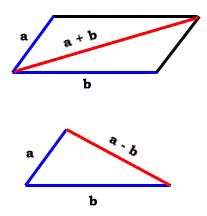Instead of projecting (a + b) as the opposite corner of a parallelogram, we simply close off the triangle of which a and b are two adjacent edges and call this triangle the area. This third edge is (a - b). Furthermore, if a and b have the same length and the angle between them is 60 degrees, then (a - b) is likewise the same length d (i.e. the triangle is equilateral), and this is the area we identify with the 2nd power of d, i.e. d x d or "d triangled". The area varies with the angle between two fixed-length vectors according to either method, as the resulting parallelogram or triangle changes shape. We will need a method to determine the angle between any two quadrays. One way to get this angle is to expand or reduce the lengths of both a and b to unity by applying a scalar of 1/distance. This will result in two vectors a' and b' with the same angle between them as between the original a and b, but both with length one: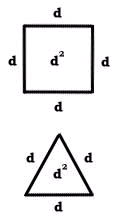```a' = a * 1/D(a) D(a') = 1 b' = b * 1/D(b) D(b') = 1 ``` We learn from Euclid that when our parallelogram is a rhombus (all sides of equal length), that (a' + b') is the perpendicular bisector of (a' - b'), which means the target angle we wish to compute breaks into two half-angles -- each part of a right triangle with an hypotenuse of length 1 and an opposite edge of (a' - b')/2. The sin( ) function accepts an angle and outputs the corresponding ratio of oppositeSide/hypotenuse, while its inverse, arcsin( ), accepts this ratio as input and outputs the angle's measure. In other words: ```sin(angle/2) = D(a' - b')/2 angle = 2 * arcsin[D(a' - b')/2] ```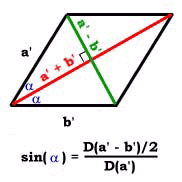One other consideration is that our native D( ) function returns its length in radians, meaning in units equaling the radius of our dense-packed ivm spheres e.g. D(2,1,1,0) = 2. However, when it comes to area and volume computations, our convention is to use the ivm sphere diameter as our unit, such that a tetrahedron defined by four ivm spheres has edge lengths of 1 and a volume of 1 (1^3 = 1). In these implementations, we use ivm sphere diameters as our units, instead of sphere radii. The function D(vector), developed in An Introduction to Quadrays, returns a vector's length or distance. Putting all of these facts together, we have enough information to design the required area methods: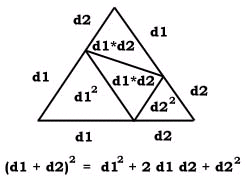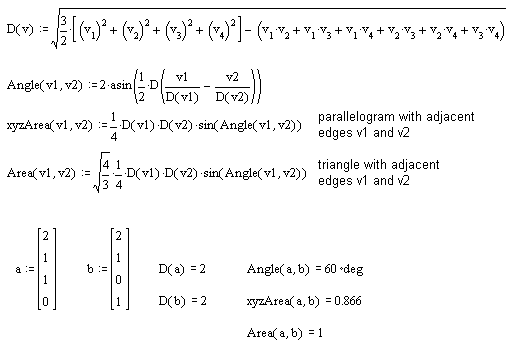The default area is equal to xyzArea multiplied by a constant, which is to be expected, since both vary linearly in proportion to edges a, b and the sine of the included angle. ```xyzArea = D(a)/2 * D(b)/2 * sin(angle) Area = D(a)/2 * D(b)/2 * sin(angle) * Root(4/3) ``` However, we should remember another important difference: xyzArea refers to the area of a parallelogram, whereas the default method refers to the area of a triangle with half the area of the corresponding parallelogram. If we halve the xyzArea, then both numbers refer to the same geometric shape and we have the basis for a conversion constant. ```Area = K * xyzArea/2 K = 2 * Area/xyzArea K = 2 * Root(4/3) ``` According to our default method, the concentric hierarchy icosahedron has an area of 20, the octahedron an area of 8, and the tetrahedron an area of 4.
 For further reading: Source code used in this study Quadrays and Volume An Introduction to Quadrays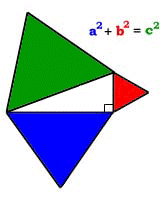Synergetics on the Web maintained by Kirby Urner